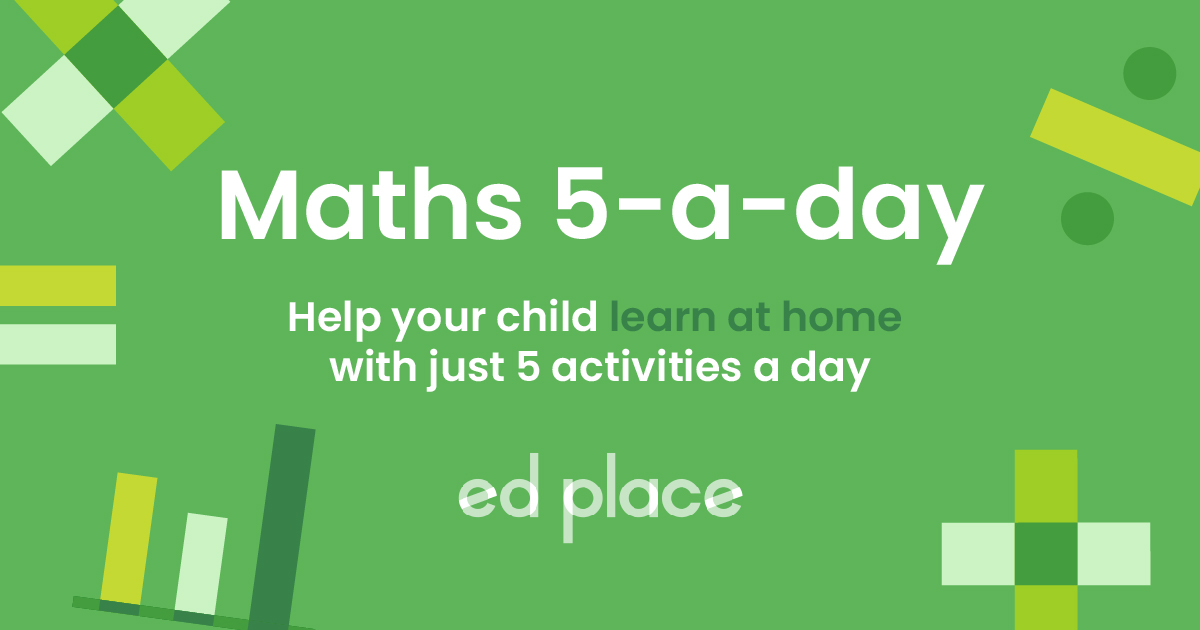# EdPlace's Year 6 Home Learning Maths Lesson: Dividing Decimals

Looking for short lessons to keep your child engaged and learning? Our experienced team of teachers have created English, maths and science lessons for the home, so your child can learn no matter where they are.  And, as all activities are self-marked, you really can encourage your child to be an independent learner. Get them started on the lesson below and then jump into our teacher-created activities to practice what they've learnt. We've recommended five to ensure they feel secure in their knowledge - 5-a-day helps keeps the learning loss at bay (or so we think!).

Are they keen to start practising straight away? Head to the bottom of the page to find the activities.

Now...onto the lesson!

Key Stage 2 Statutory Requirements for Maths
Year 6 students should be taught to use written division methods in cases where the answer has up to 2 decimal places.

# Dividing decimals with up to two decimal places

Dividing decimals is really useful for day-to-day life. For example, when using money, converting measurements and making a recipe smaller/larger. Dividing decimals is actually as simple as dividing whole numbers – you just need to know how to use the ‘bus stop’ method!

Every single lesson taught in school has an objective that each child should achieve. We are confident that by the end of reading this you and your child will:

1) Understand how to divide decimals with up to 2 decimal places by a whole number

2) Apply this to independent work

3) Explain how they completed their work back to you!

## Step 1 - Understand key terminology

The dividend is the number you are dividing by – the larger number.

The divisor is the number you are dividing into that larger number – the smaller number.

The ‘bus stop method’ is the way we lay out the question – it looks like a bus stop (see below)!This shows 66 ÷ 2 = 33

The remainder is the number left over if a number doesn’t multiply exactly into another.

The decimal point is the dot after the ones column (see Step 2 for an example) – it’s important to remember this because it shows that the number(s) after the decimal point are only part of a whole number.

A decimal place is one of the columns after the decimal point – one decimal place is the tenths column, two decimal places is the hundredths column (see Step 2 for an example).

A place holder is the number 0 to show there is nothing in that column.

## Step 2 - Check your child's prior understanding!

Your child will need to know their times tables so they can do the opposite to divide.

They will also need to have a basic understanding of decimal place value and the names of the columns:## Step 3 - The bus stop method

To divide a decimal by a whole number, you need to write the dividend inside the ‘bus stop’. The divisor goes outside of the ‘bus stop’:1) This says 53.4 ÷ 3

Now, you work out how many 3s go into 5 (5 ÷ 3).

The answer is 1 remainder 2.

Write the 1 above the 5. Carry the 2 to the next number.

Now, you work out 23 ÷ 3. The answer is 7 remainder 2.

Write the 7 above the 3. Carry the 2 to the next digit along.

Now, you work out 24 ÷ 3. The answer is 8.

Write the 8 above the 4. There is no remainder.

But wait – don't forget to put the decimal point in or the answer will be wrong.2) Let’s do another example together:

12.98 ÷ 4

We can’t do 1 ÷ 4 so we carry the 1 to the next digit.

Now we do 12 ÷ 4, which is 3.

We write three above the 2.

There is no remainder to carry.

9 ÷ 4 = 2 with 1 remaining.

Write the 2 above the 9 and carry the 1 to the next digit.

18 ÷ 4 = 4. There is 2 remaining, so what to do now…?We put a 0 in the dividend (the decimal number) next to the 8 – this acts as a place holder to show there are no thousandths.

Now, we can carry the remaining 2 along to the 0.

20 ÷ 4 = 5.

Put the 5 above the 0 and there is no remainder to carry.

Don’t forget to put the decimal point in!## Step 4 - Putting it into practise...

Now have a go at these examples together:

a) 17.25 ÷ 5

b) 26.44 ÷ 4

Challenge questions:

c) 49.24 ÷ 8

d) 29.46 ÷ 4

## Step 5 - Give it a go...

Now that you’ve covered how to divide decimals together, why not put this to the test and assign your child the following activities in this order? All activities are created by teachers and automatically marked. Plus, with an EdPlace subscription, we can automatically progress your child at a level that's right for them. Sending you progress reports along the way so you can track and measure progress, together - brilliant!

a) 3.45

b) 6.61

c) 6.155

d) 7.365

Keep going! Looking for more activities, different subjects or year groups?

Click the button below to view the EdPlace English, maths, science and 11+ activity library